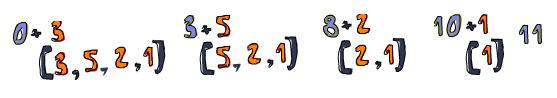# Blog

## Functional programming in Python: a little bit more

In our first blog post of functional programing in Python, we talked about the main concepts and showed the most important functional constructs in Python. For this blog post I will assume you already read the first part, because we will go deeper into some concepts now. Bear in mind that all examples that follow will be provided in Python3.

This blog entry has two main goals. The first one is to help you understand what is function composition. In the second goal, we will deal with two of the best Python modules: operator and functools, which can be used to construct better high-order functions and will also help you to do function composition. That way we can easily combine functions we already built, leaving code more declarative. Let's see how.

# Function Composition made easy

Before we start, let me explain a little about function composition. To say it simple it is when your function is composed by other functions internally.

Take a look at the code below:

```def clean_and_lower(text):
return text.strip().lower()

print(clean_and_lower("Anderson ")) # prints anderson
```

The function `clean_and_lower` is composed by both `strip` and `lower` functions. First the text is striped off blanks and then the result is showed in lower case.

Let's write an function to help us to do a simple composition:

```def compose(f1, f2):
return lambda x: f1(f2(x)) # returns a new funcion composed by the functions passed as argument
```

Now, we can use that function as follows:

```clean_and_lower = compose(str.lower, str.strip) # returns the composed function
print(clean_and_lower("Anderson ")) # also prints anderson
```

Think of it as if we were plaing with Lego. There are blocks that we can put together to do something more complex. By using function composition we can combine a lot of functions into other functions and so, the code becomes more declarative. This is one of the main concepts in functional programming.

# Operator: contributing towards the use of high-order functions

The operator module provides a lot of functions to perform basic operations that can be used isolated or combined with other functions.

Check the code below:

```result = 1 + 2
print(result) # prints 3

import operator
result = operator.add(1, 2)
print(result) # prints 3 too, operator.add is only a function that performs addition

print('A'+'B') # prints AB
print(operator.concat("A", "B")) # prints AB too
```

There are a lot of other functions like `operator.eq`, `operator.gt` and others. I know that you may be wondering why one would use operator instead of simply using `1 + 2`. I will show you when to use this module and why it's waaay better to use operator as High-order functions.

# Functools, a walk in the park

Let’s start showing the `reduce` function, that receives one function and a array of values and combine them together, returning a single value. Remember to import the functools module.

```import functools
```

Let’s sum a list of numbers:

```print(functools.reduce(lambda a, b: a+b, [3, 5, 2, 1], 0)) # prints 11
```

In the example above the lambda function receives two values and sums them. The `reduce` function iterates the list while applying the function, combining results until a single `11` value is computed.

Look the image below to understand how `reduce` works:Take a look now how we can replace the `lambda` function by using the `operator.add`.

```print(functools.reduce(operator.add, [3, 5, 2, 1], 0)) # prints 11
```

Easy peasy, right? The code is simpler and more declarative using `operator.add`.

Let’s use again with the `operator.concat`:

```print(functools.reduce(operator.concat, ["Reduce", " is", " Awesome!"])) # prints Reduce is Awesome
```

Other important function is `partial`, which is also present in the functools module. The partial function is used to facilitate the composition of other functions.

Check the example below using the `operator.concat`:

```call_me_mr = functools.partial(operator.concat, "Mr ")
call_me_mrs = functools.partial(operator.concat, "Mrs ")
```

The `partial` function receives one function, in the case above the `operator.concat`. One second argument is passed, in our case, the value `Mr`. In that way we get back another function that expects the second value of the `operator.concat`.

```print(call_me_mr("Anderson")) # prints Mr Anderson
print(call_me_mrs("Renata")) # prints Mrs Renata
```

See? You can interpret `call_me_mr` as a partial application of `operator.concat`. It's partial because it's missing the second argument, which we pass later by calling `call_me_mr`.

Let’s see an example a little bit more interesting of the use of `partial`. We'll combine partial with the `sorted` function and the `operator.attrgetter` function:

```person = namedtuple('Person', 'name age')
p1 = person('Anderson', 29)
p2 = person('Renato', 25)
p3 = person('Diego', 27)

persons = [p1, p2, p3] # a list of persons

sort_by_name = functools.partial(sorted, key=lambda t: t.name) # returns one function that order by name
sort_by_age = functools.partial(sorted, key=lambda t: t.age) # returns one function that order by age

print(sort_by_name(persons)) # prints Anderson, Diego and Renato
print(sort_by_age(persons)) # prints Renato, Diego, Anderson
```

How about replacing those lambda functions for `operator.attrgetter`?

```sort_by_name = functools.partial(sorted, key=operator.attrgetter('name'))
sort_by_age = functools.partial(sorted, key=operator.attrgetter('age'))
```

We get the same result with a more declarative code.

# Sum-up

We introduced some intermediate functional programming concepts, as partial and function composition, applying them using Python. Challenge yourself to see in which moments you can use partial, doing function composition. You will see how this is cool. Also check by yourself the functools and operator modules.

# References